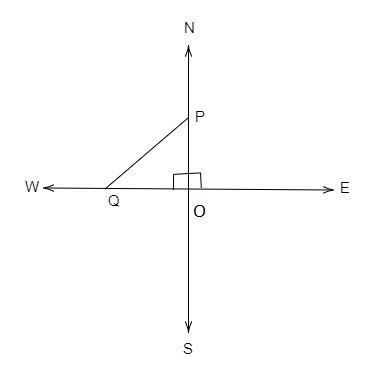# An aeroplane leaves an airport and flies due north at a speed of 1000 km per hour. At the same time, another aeroplane leaves the same airport and flies due west at a speed of 1200 km per hour. How far apart will be the two planes after $1\frac{1}{2}$ hours?

Given:

An aeroplane leaves an airport and flies due north at a speed of 1,000 km/hr. At the same time, another aeroplane leaves the same airport and flies due west at a speed of 1,200 km/hr.

To do:

We have to find the distance between the two planes after $1\frac{1}{2}$ hours.

Solution:Speed of the first aeroplane $=1000\ km/hr$

Time $=1\frac{1}{2}\ hr=\frac{2\times1+1}{2}\ hr=\frac{3}{2}\ hr$

Distance travelled by the first aeroplane$=Speed\times Time$

$OP=1000\times\frac{3}{2}\ km$

$=500\times3\ km$

$=1500\ km$

The final position of the first aeroplane is represented by point P in the figure.

Speed of the second aeroplane $=1200\ km/hr$

Time $=1\frac{1}{2}\ hr=\frac{2\times1+1}{2}\ hr=\frac{3}{2}\ hr$

Distance travelled by the second aeroplane$=Speed\times Time$

$OQ=1200\times\frac{3}{2}\ km$

$=600\times3\ km$

$=1800\ km$

The final position of the second aeroplane is represented by point Q in the figure.

The distance between the aeroplanes is represented by PQ in the figure.

$\triangle OPQ$ is a right-angled triangle. By Pythagoras theorem,

$PQ^2=OP^2+OQ^2$

$PQ^2=(1500)^2+(1800)^2$

$PQ^2=2250000+3240000$

$PQ^2=5490000$

$PQ=\sqrt{5490000}$

$PQ=\sqrt{90000\times61}$

$PQ=300\sqrt{61}\ km$

The two planes will be $300\sqrt{61}\ km$ apart after $1\frac{1}{2}$ hours.

Updated on: 10-Oct-2022

62 Views